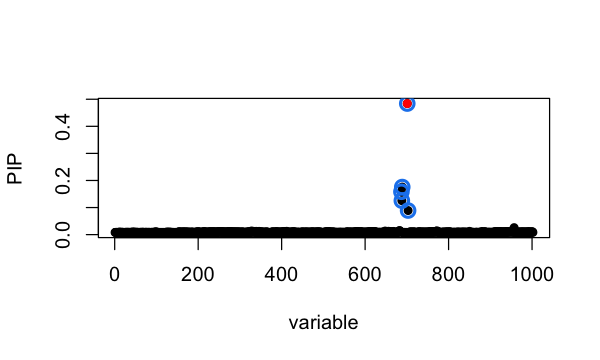Diagnostic for fine-mapping with summary statistics

2021-11-12

This vignette demonstrates diagnostic plot for consistency between summary statistics and reference LD matrix.

The susie_rss assumes the LD matrix accurately estimate the correlations among SNPs from the original GWAS genotype data. Typically, the LD matrix comes from some public database of genotypes in a suitable reference population. The inaccurate LD information leads to unreliable fine-mapping result.

The diagnostic for consistency between summary statistics and refenrence LD matrix is based on the RSS model under the null with regularized LD matrix. $\hat{z} | R, s \sim N(0, (1-s)R + s I), 0<s<1$ The parameter s is estimated by maximum likelihood. A larger s means a greater inconsistency between summary statistics and the LD matrix. The expected z score is computed for each SNP, $$E(\hat{z}_j | \hat{z}_{-j})$$, and plotted against the observed z scores.

library(susieR)
library(curl)

LD information from the original genotype data

We demonstrate the diagnostic plot in a simple case, the LD matrix is estimated from the original genotype data. We use the same simulated data as in fine mapping vignette.

data("N3finemapping")
b = N3finemapping$true_coef[,1] sumstats <- univariate_regression(N3finemapping$X, N3finemapping$Y[,1]) z_scores <- sumstats$betahat / sumstats$sebetahat Rin = cor(N3finemapping$X)
attr(Rin, "eigen") = eigen(Rin, symmetric = T)
susie_plot(z_scores, y = "z", b=b)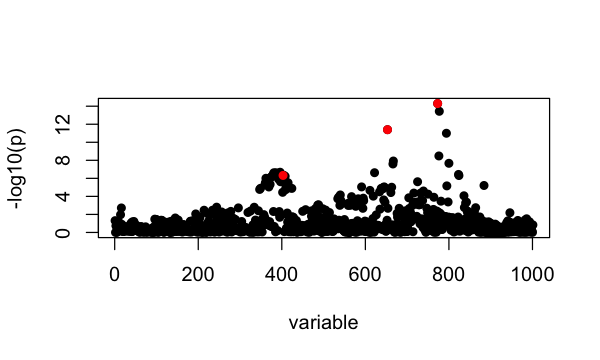The estimated s is

s
#  0.0001123485

The plot for the observed z scores vs the expected z scores is

condz_in$plot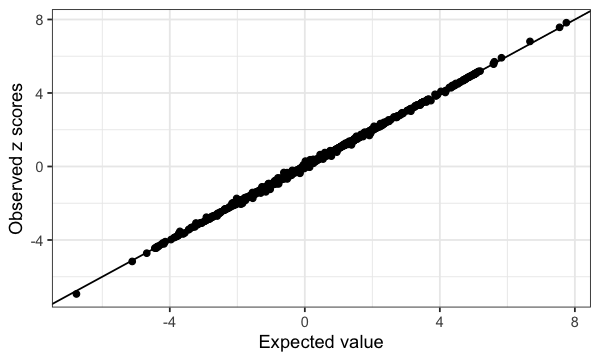Summary of SuSiE Credible Sets: fit <- susie_rss(z_scores, Rin) susie_plot(fit,y = "PIP", b=b)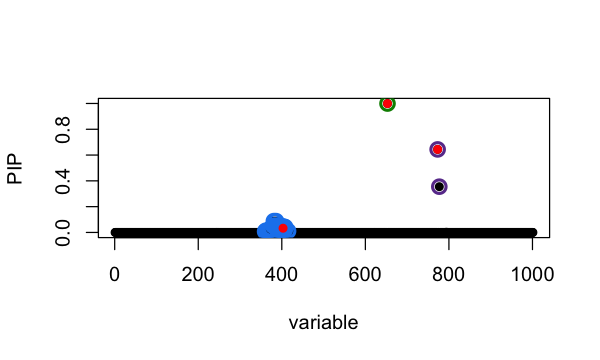LD information from the reference panel We use another simulated data where the LD matrix is estimated from a reference panel. There is one signal in the simulated data (red point). There is one SNP with mismatched reference and alternative allele between summary statistics and the reference panel (yellow point). data_file <- tempfile(fileext = ".RData") data_url <- paste0("https://raw.githubusercontent.com/stephenslab/susieR/", "master/inst/datafiles/SummaryConsistency1k.RData") curl_download(data_url,data_file) load(data_file) zflip = SummaryConsistency$z
ld = SummaryConsistency$ldref b = numeric(length(zflip)) b[SummaryConsistency$signal_id] = zflip[SummaryConsistency$signal_id] plot(zflip, pch = 16, col = "#767676", main = "Marginal Associations", xlab="SNP", ylab = "z scores") points(SummaryConsistency$signal_id, zflip[SummaryConsistency$signal_id], col=2, pch=16) points(SummaryConsistency$flip_id, zflip[SummaryConsistencyflip_id], col=7, pch=16)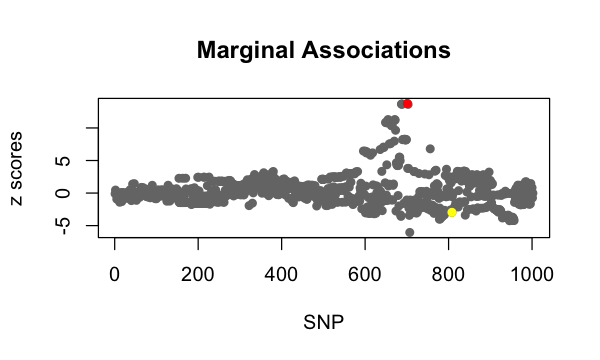Using the data with misaligned allele, SuSiE-RSS identifies a true positive CS containing the true effect SNP; and a false positive CS that incorrectly contains the mismatched SNP. fit = susie_rss(zflip, ld) susie_plot(fit, y='PIP', b=b) points(SummaryConsistencyflip_id, fit$pip[SummaryConsistency$flip_id], col=7, pch=16)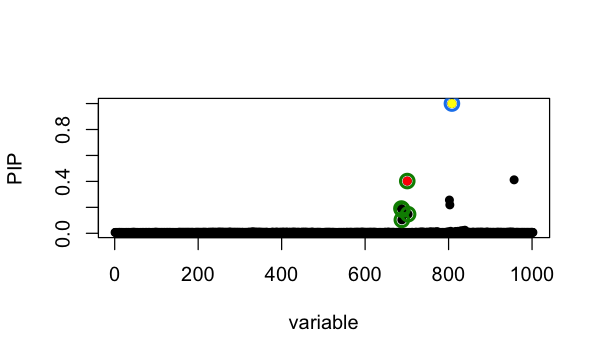The estimated s is

condz$plot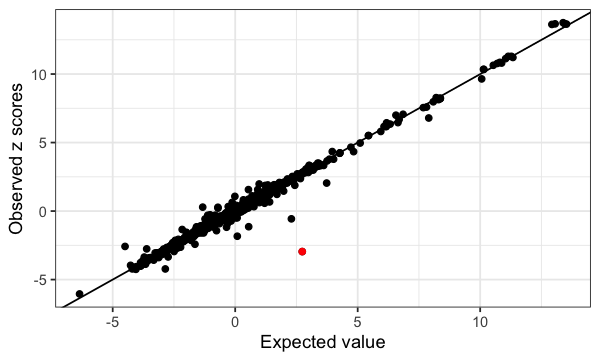After fixing the allele encoding, SuSiE-RSS identifies a single true positive CS containing the true-effect SNP, and the formerly mismatched SNP is (correctly) not included in a CS. z = zflip z[SummaryConsistency$flip_id] = -z[SummaryConsistency\$flip_id]